Note

This page is a reference documentation. It only explains the class signature, and not how to use it. Please refer to the user guide for the big picture.

# nilearn.glm.first_level.FirstLevelModel#

class nilearn.glm.first_level.FirstLevelModel(t_r=None, slice_time_ref=0.0, hrf_model='glover', drift_model='cosine', high_pass=0.01, drift_order=1, fir_delays=, min_onset=-24, mask_img=None, target_affine=None, target_shape=None, smoothing_fwhm=None, memory=Memory(location=None), memory_level=1, standardize=False, signal_scaling=0, noise_model='ar1', verbose=0, n_jobs=1, minimize_memory=True, subject_label=None, random_state=None)[source]#

Implement the General Linear Model for single session fMRI data.

Parameters:
t_rfloat

This parameter indicates repetition times of the experimental runs. In seconds. It is necessary to correctly consider times in the design matrix. This parameter is also passed to `nilearn.signal.clean`. Please see the related documentation for details.

slice_time_reffloat, default=0

This parameter indicates the time of the reference slice used in the slice timing preprocessing step of the experimental runs. It is expressed as a fraction of the `t_r` (repetition time), so it can have values between 0. and 1.

hrf_model`str`, function, list of functions, or None

This parameter defines the HRF model to be used. It can be a string if you are passing the name of a model implemented in Nilearn. Valid names are:

It can also be a custom model. In this case, a function should be provided for each regressor. Each function should behave as the other models implemented within Nilearn. That is, it should take both t_r and oversampling as inputs and return a sample numpy array of appropriate shape.

Note

It is expected that “spm” standard and “glover” models would not yield large differences in most cases.

Note

In case of “glover” and “spm” models, the derived regressors are orthogonalized with respect to the main one.

Default=’glover’.

drift_modelstring, default=’cosine’

This parameter specifies the desired drift model for the design matrices. It can be ‘polynomial’, ‘cosine’ or None.

high_passfloat, default=0.01

This parameter specifies the cut frequency of the high-pass filter in Hz for the design matrices. Used only if drift_model is ‘cosine’.

drift_orderint, default=1

This parameter specifies the order of the drift model (in case it is polynomial) for the design matrices.

fir_delaysarray of shape(n_onsets) or list, default=

In case of FIR design, yields the array of delays used in the FIR model, in scans.

min_onsetfloat, default=-24

This parameter specifies the minimal onset relative to the design (in seconds). Events that start before (slice_time_ref * t_r + min_onset) are not considered.

Mask to be used on data. If an instance of masker is passed, then its mask will be used. If no mask is given, it will be computed automatically by a NiftiMasker with default parameters. If False is given then the data will not be masked.

target_affine3x3 or 4x4 matrix, optional

This parameter is passed to nilearn.image.resample_img. Please see the related documentation for details.

target_shape3-tuple of integers, optional

This parameter is passed to nilearn.image.resample_img. Please see the related documentation for details.

smoothing_fwhm`float`, optional.

If smoothing_fwhm is not None, it gives the full-width at half maximum in millimeters of the spatial smoothing to apply to the signal.

memorystring or pathlib.Path, optional

Path to the directory used to cache the masking process and the glm fit. By default, no caching is done. Creates instance of joblib.Memory.

memory_levelinteger, optional

Rough estimator of the amount of memory used by caching. Higher value means more memory for caching.

standardizeboolean, default=False

If standardize is True, the time-series are centered and normed: their variance is put to 1 in the time dimension.

signal_scalingFalse, int or (int, int), default=0

If not False, fMRI signals are scaled to the mean value of scaling_axis given, which can be 0, 1 or (0, 1). 0 refers to mean scaling each voxel with respect to time, 1 refers to mean scaling each time point with respect to all voxels & (0, 1) refers to scaling with respect to voxels and time, which is known as grand mean scaling. Incompatible with standardize (standardize=False is enforced when signal_scaling is not False).

noise_model{‘ar1’, ‘ols’}, default=’ar1’

The temporal variance model.

verboseinteger, default=0

Indicate the level of verbosity. By default, nothing is printed. If 0 prints nothing. If 1 prints progress by computation of each run. If 2 prints timing details of masker and GLM. If 3 prints masker computation details.

n_jobsinteger, default=1

The number of CPUs to use to do the computation. -1 means ‘all CPUs’, -2 ‘all CPUs but one’, and so on.

minimize_memoryboolean, default=True

Gets rid of some variables on the model fit results that are not necessary for contrast computation and would only be useful for further inspection of model details. This has an important impact on memory consumption.

subject_labelstring, optional

This id will be used to identify a FirstLevelModel when passed to a SecondLevelModel object.

random_stateint or numpy.random.RandomState, default=None.

Random state seed to sklearn.cluster.KMeans for autoregressive models of order at least 2 (‘ar(N)’ with n >= 2).

New in version 0.9.1.

Attributes:
labels_array of shape (n_voxels,),

a map of values on voxels used to identify the corresponding model

results_dict,

with keys corresponding to the different labels values. Values are SimpleRegressionResults corresponding to the voxels, if minimize_memory is True, RegressionResults if minimize_memory is False

__init__(t_r=None, slice_time_ref=0.0, hrf_model='glover', drift_model='cosine', high_pass=0.01, drift_order=1, fir_delays=, min_onset=-24, mask_img=None, target_affine=None, target_shape=None, smoothing_fwhm=None, memory=Memory(location=None), memory_level=1, standardize=False, signal_scaling=0, noise_model='ar1', verbose=0, n_jobs=1, minimize_memory=True, subject_label=None, random_state=None)[source]#
property scaling_axis#

Return scaling of axis.

fit(run_imgs, events=None, confounds=None, sample_masks=None, design_matrices=None, bins=100)[source]#

Fit the GLM.

For each run: 1. create design matrix X 2. do a masker job: fMRI_data -> Y 3. fit regression to (Y, X)

Parameters:
run_imgsNiimg-like object or list of Niimg-like objects,

Data on which the GLM will be fitted. If this is a list, the affine is considered the same for all.

eventspandas Dataframe or string or list of pandas DataFrames or strings, default=None

fMRI events used to build design matrices. One events object expected per run_img. Ignored in case designs is not None. If string, then a path to a csv file is expected.

confoundspandas Dataframe, numpy array or string or list of pandas DataFrames, numpy arrays or strings, default=None

Each column in a DataFrame corresponds to a confound variable to be included in the regression model of the respective run_img. The number of rows must match the number of volumes in the respective run_img. Ignored in case designs is not None. If string, then a path to a csv file is expected.

sample_masksarray_like, or list of array_like, default=None

shape of array: (number of scans - number of volumes remove) Indices of retained volumes. Masks the niimgs along time/fourth dimension to perform scrubbing (remove volumes with high motion) and/or remove non-steady-state volumes.

New in version 0.9.2.

design_matricespandas DataFrame or list of pandas DataFrames, default=None

Design matrices that will be used to fit the GLM. If given it takes precedence over events and confounds.

binsint, default=100

Maximum number of discrete bins for the AR coef histogram. If an autoregressive model with order greater than one is specified then adaptive quantification is performed and the coefficients will be clustered via K-means with bins number of clusters.

compute_contrast(contrast_def, stat_type=None, output_type='z_score')[source]#

Generate different outputs corresponding to the contrasts provided e.g. z_map, t_map, effects and variance.

In multi-session case, outputs the fixed effects map.

Parameters:
contrast_defstr or array of shape (n_col) or list of (string or

array of shape (n_col))

where `n_col` is the number of columns of the design matrix, (one array per run). If only one array is provided when there are several runs, it will be assumed that the same contrast is desired for all runs. One can use the name of the conditions as they appear in the design matrix of the fitted model combined with operators +- and combined with numbers with operators +-*/. In this case, the string defining the contrasts must be a valid expression for compatibility with `pandas.DataFrame.eval`.

stat_type{‘t’, ‘F’}, optional

Type of the contrast.

output_typestr, default=’z_score’

Type of the output map. Can be ‘z_score’, ‘stat’, ‘p_value’, ‘effect_size’, ‘effect_variance’ or ‘all’.

Returns:
outputNifti1Image or dict

The desired output image(s). If `output_type == 'all'`, then the output is a dictionary of images, keyed by the type of image.

fit_transform(X, y=None, **fit_params)#

Fit to data, then transform it.

Fits transformer to X and y with optional parameters fit_params and returns a transformed version of X.

Parameters:
Xarray-like of shape (n_samples, n_features)

Input samples.

yarray-like of shape (n_samples,) or (n_samples, n_outputs), default=None

Target values (None for unsupervised transformations).

**fit_paramsdict

Returns:
X_newndarray array of shape (n_samples, n_features_new)

Transformed array.

generate_report(contrasts, title=None, bg_img='MNI152TEMPLATE', threshold=3.09, alpha=0.001, cluster_threshold=0, height_control='fpr', min_distance=8.0, plot_type='slice', display_mode=None, report_dims=(1600, 800))[source]#

Return a `HTMLReport` which shows all important aspects of a fitted GLM.

The `HTMLReport` can be opened in a browser, displayed in a notebook, or saved to disk as a standalone HTML file.

The GLM must be fitted and have the computed design matrix(ces).

Note

The `FirstLevelModel` or `SecondLevelModel` must have been fitted prior to calling `generate_report`.

Parameters:
contrasts

Contrasts information for a `FirstLevelModel` or `SecondLevelModel`.

Example:

Dict of contrast names and coefficients, or list of contrast names or list of contrast coefficients or contrast name or contrast coefficient

Each contrast name must be a string. Each contrast coefficient must be a list or numpy array of ints.

Contrasts are passed to `contrast_def` for `FirstLevelModel` through `compute_contrast`, and `second_level_contrast` for `SecondLevelModel` through `compute_contrast`.

title`str`, optional
• If a `str`, it represents the web page’s title and primary heading, model type is sub-heading.

• If `None`, page titles and headings are autogenerated using contrast names.

bg_imgNiimg-like object, default=’MNI152TEMPLATE’

See Input and output: neuroimaging data representation. The background image for mask and stat maps to be plotted on upon. To turn off background image, just pass “bg_img=None”.

threshold`float`, default=3.09

Cluster forming threshold in same scale as `stat_img` (either a t-scale or z-scale value). Used only if `height_control` is `None`.

alpha`float`, default=0.001

Number controlling the thresholding (either a p-value or q-value). Its actual meaning depends on the `height_control` parameter. This function translates alpha to a z-scale threshold.

cluster_threshold`int`, default=0

Cluster size threshold, in voxels.

height_control`str` or None, default=’fpr’

False positive control meaning of cluster forming threshold: ‘fpr’, ‘fdr’, ‘bonferroni’ or `None`.

min_distance`float`, default=8.0

For display purposes only. Minimum distance between subpeaks in mm.

plot_type{‘slice’, ‘glass’}, default=’slice’

Specifies the type of plot to be drawn for the statistical maps.

display_mode{‘ortho’, ‘x’, ‘y’, ‘z’, ‘xz’, ‘yx’, ‘yz’, ‘l’, ‘r’, ‘lr’, ‘lzr’, ‘lyr’, ‘lzry’, ‘lyrz’}, optional

Choose the direction of the cuts:

• ‘x’ - sagittal

• ‘y’ - coronal

• ‘z’ - axial

• ‘l’ - sagittal left hemisphere only

• ‘r’ - sagittal right hemisphere only

• ‘ortho’ - three cuts are performed in orthogonal directions

Default is ‘z’ if `plot_type` is ‘slice’; ‘ortho’ if `plot_type` is ‘glass’.

report_dimsSequence[ `int`, `int` ], default=(1600, 800)

Specifies width, height (in pixels) of report window within a notebook. Only applicable when inserting the report into a Jupyter notebook. Can be set after report creation using `report.width`, `report.height`.

Returns:
report_text`HTMLReport`

Contains the HTML code for the GLM report.

Get metadata routing of this object.

Please check User Guide on how the routing mechanism works.

Returns:

A `MetadataRequest` encapsulating routing information.

get_params(deep=True)#

Get parameters for this estimator.

Parameters:
deepbool, default=True

If True, will return the parameters for this estimator and contained subobjects that are estimators.

Returns:
paramsdict

Parameter names mapped to their values.

predicted()[source]#

Transform voxelwise predicted values to the same shape as the input Nifti1Image(s).

Returns:
outputlist

A list of Nifti1Image(s).

r_square()[source]#

Transform voxelwise r-squared values to the same shape as the input Nifti1Image(s).

Returns:
outputlist

A list of Nifti1Image(s).

residuals()[source]#

Transform voxelwise residuals to the same shape as the input Nifti1Image(s).

Returns:
outputlist

A list of Nifti1Image(s).

set_fit_request(*, bins='\$UNCHANGED\$', confounds='\$UNCHANGED\$', design_matrices='\$UNCHANGED\$', events='\$UNCHANGED\$', run_imgs='\$UNCHANGED\$', sample_masks='\$UNCHANGED\$')#

Request metadata passed to the `fit` method.

Note that this method is only relevant if `enable_metadata_routing=True` (see `sklearn.set_config`). Please see User Guide on how the routing mechanism works.

The options for each parameter are:

• `True`: metadata is requested, and passed to `fit` if provided. The request is ignored if metadata is not provided.

• `False`: metadata is not requested and the meta-estimator will not pass it to `fit`.

• `None`: metadata is not requested, and the meta-estimator will raise an error if the user provides it.

• `str`: metadata should be passed to the meta-estimator with this given alias instead of the original name.

The default (`sklearn.utils.metadata_routing.UNCHANGED`) retains the existing request. This allows you to change the request for some parameters and not others.

New in version 1.3.

Note

This method is only relevant if this estimator is used as a sub-estimator of a meta-estimator, e.g. used inside a `Pipeline`. Otherwise it has no effect.

Parameters:
binsstr, True, False, or None, default=sklearn.utils.metadata_routing.UNCHANGED

Metadata routing for `bins` parameter in `fit`.

confoundsstr, True, False, or None, default=sklearn.utils.metadata_routing.UNCHANGED

Metadata routing for `confounds` parameter in `fit`.

design_matricesstr, True, False, or None, default=sklearn.utils.metadata_routing.UNCHANGED

Metadata routing for `design_matrices` parameter in `fit`.

eventsstr, True, False, or None, default=sklearn.utils.metadata_routing.UNCHANGED

Metadata routing for `events` parameter in `fit`.

run_imgsstr, True, False, or None, default=sklearn.utils.metadata_routing.UNCHANGED

Metadata routing for `run_imgs` parameter in `fit`.

Metadata routing for `sample_masks` parameter in `fit`.

Returns:
selfobject

The updated object.

set_output(*, transform=None)#

Set output container.

See Introducing the set_output API for an example on how to use the API.

Parameters:
transform{“default”, “pandas”}, default=None

Configure output of transform and fit_transform.

• “default”: Default output format of a transformer

• “pandas”: DataFrame output

• None: Transform configuration is unchanged

Returns:
selfestimator instance

Estimator instance.

set_params(**params)#

Set the parameters of this estimator.

The method works on simple estimators as well as on nested objects (such as `Pipeline`). The latter have parameters of the form `<component>__<parameter>` so that it’s possible to update each component of a nested object.

Parameters:
**paramsdict

Estimator parameters.

Returns:
selfestimator instance

Estimator instance.

## Examples using `nilearn.glm.first_level.FirstLevelModel`#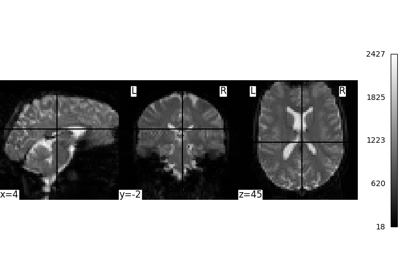Intro to GLM Analysis: a single-session, single-subject fMRI dataset

Intro to GLM Analysis: a single-session, single-subject fMRI dataset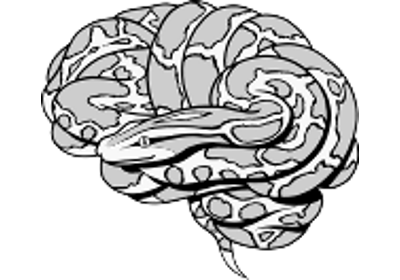Decoding of a dataset after GLM fit for signal extraction

Decoding of a dataset after GLM fit for signal extractionExample of explicit fixed effects fMRI model fitting

Example of explicit fixed effects fMRI model fitting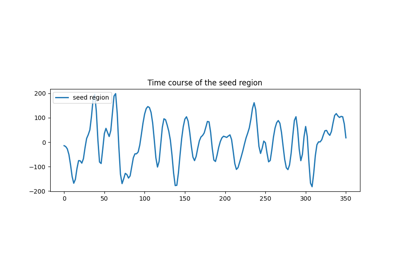Default Mode Network extraction of ADHD dataset

Default Mode Network extraction of ADHD dataset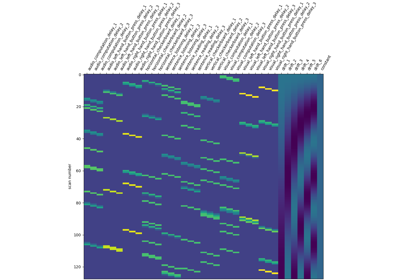Analysis of an fMRI dataset with a Finite Impule Response (FIR) model

Analysis of an fMRI dataset with a Finite Impule Response (FIR) model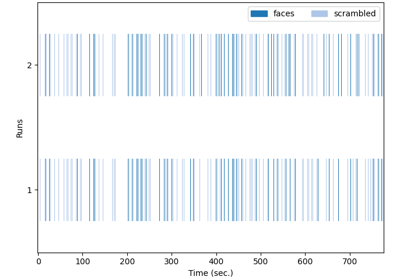Single-subject data (two sessions) in native space

Single-subject data (two sessions) in native spaceSimple example of two-session fMRI model fitting

Simple example of two-session fMRI model fitting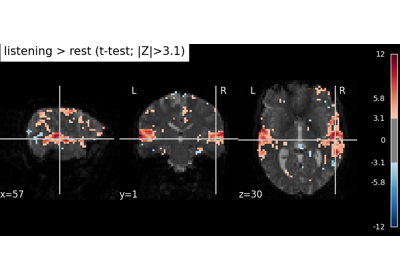Predicted time series and residuals

Predicted time series and residuals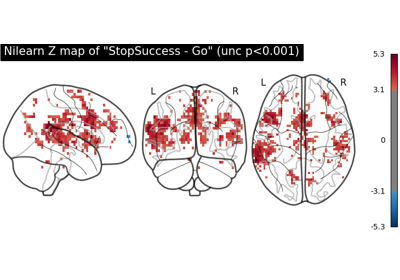First level analysis of a complete BIDS dataset from openneuro

First level analysis of a complete BIDS dataset from openneuro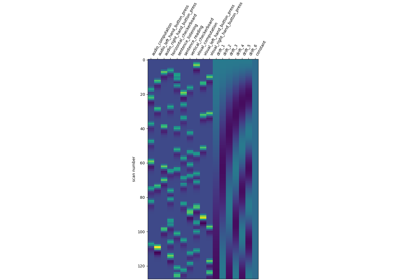Understanding parameters of the first-level model

Understanding parameters of the first-level model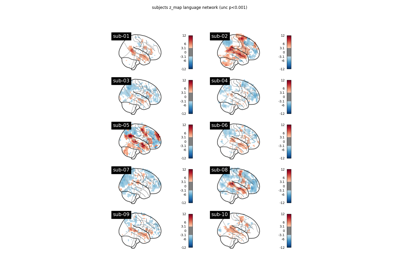BIDS dataset first and second level analysis

BIDS dataset first and second level analysis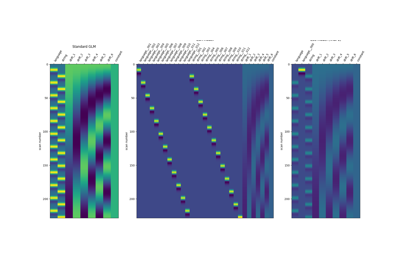Beta-Series Modeling for Task-Based Functional Connectivity and Decoding

Beta-Series Modeling for Task-Based Functional Connectivity and Decoding